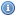# Number Theory Books, Documents, and Lectures

 Title Year DescriptionA Course In Algebraic Number Theory 2003An introduction to the subject, covering both global and local fields. The prerequisite is a standard graduate course in algebra.A Computational Introduction to Number Theory and Algebra 2005A book introducing basic concepts from computational number theory and algebra, including all the necessary mathematical background.An Introduction to the Theory of Numbers 2004These lectures are intended as an introduction to the elementary theory of numbers.